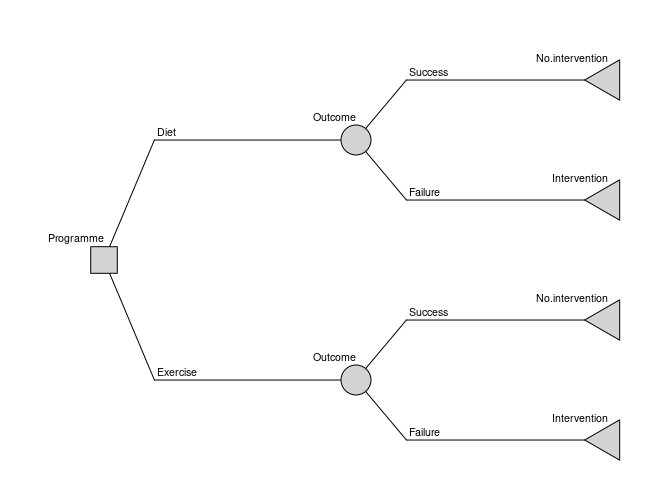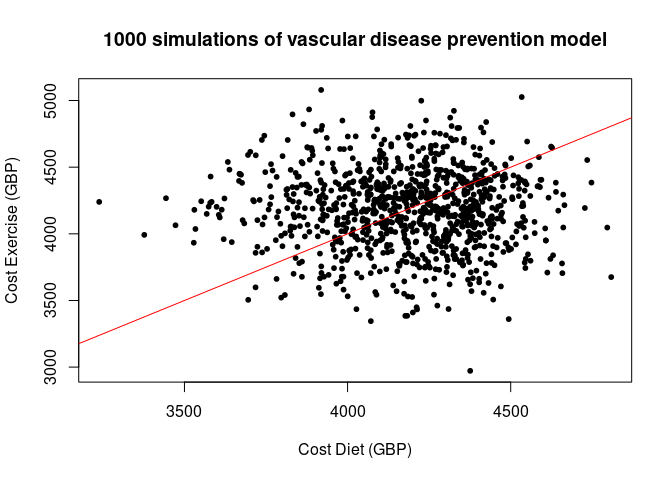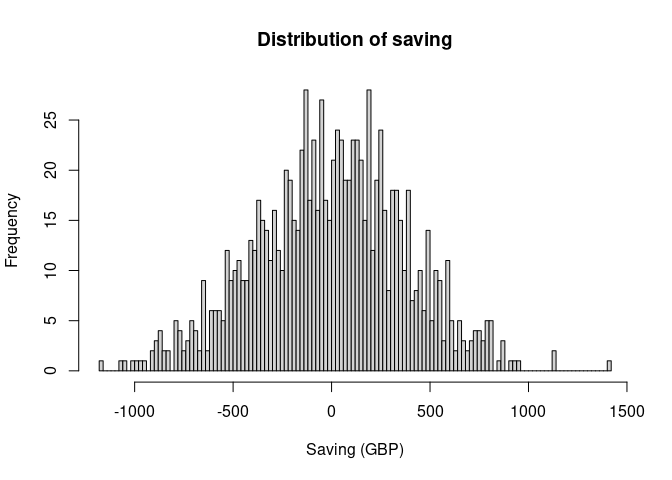# Decision Tree representations

A decision tree is a decision model that represents all possible pathways through sequences of events (nodes), which can be under the experimenter’s control (decisions) or not (chances). A decision tree can be represented visually according to a standardised grammar:

• Decision nodes (represented graphically by a square $$\square$$): these represent alternative paths that the model should compare, for example different treatment plans. Each Decision node must be the source of two or more Actions. A decision tree can have one or more Decision nodes, which determine the possible strategies that the model compares.
• Chance nodes (represented graphically by a circle $$\bigcirc$$): these represent alternative paths that are out of the experiment’s control, for example the probability of developing a certain side effect. Each Chance node must be the source of one or more Reactions, each with a specified probability. The probability of Reactions originating from a single Chance node must sum to 1.
• Leaf nodes (represented graphically by a triangle $$\lhd$$): these represent the final outcomes of a path. No further Actions or Reactions can occur after a Leaf node. A Leaf node can have a utility value (to a maximum of 1, indicating perfect utility) and an interval over which the utility applies.

• Actions arise from Decision nodes, and
• Reactions arise from Chance nodes.

rdecision builds a Decision Tree model by defining these elements and their relationships. For example, consider the fictitious and idealized decision problem, introduced in the package README file, of choosing between providing two forms of lifestyle advice, offered to people with vascular disease, which reduce the risk of needing an interventional procedure. The cost to a healthcare provider of the interventional procedure (e.g. inserting a stent) is 5000 GBP; the cost of providing the current form of lifestyle advice, an appointment with a dietician (“diet”), is 50 GBP and the cost of providing an alternative form, attendance at an exercise programme (“exercise”), is 750 GBP. If the advice programme is successful, there is no need for an interventional procedure.

The model for this fictional scenario can be defined by the following elements:

• Decision node: which programme to enrol the patient in.
decision_node <- DecisionNode$new("Programme") • Chance nodes: the chance that the patient will need an interventional procedure. This is different for the two programmes, so two chance nodes must be defined. chance_node_diet <- ChanceNode$new("Outcome")
chance_node_exercise <- ChanceNode$new("Outcome") • Leaf nodes: the possible final states of the model, depending both on the decision (which programme) and the chance of needing an intervention. Here, we assume that the model has a time horizon of 1 year, and that the utility is the same for all patients (the default values). leaf_node_diet_no_stent <- LeafNode$new("No intervention")
leaf_node_diet_stent <- LeafNode$new("Intervention") leaf_node_exercise_no_stent <- LeafNode$new("No intervention")
leaf_node_exercise_stent <- LeafNode$new("Intervention") These nodes can then be wired into a decision tree graph by defining the edges that link pairs of nodes: • Actions: the two programmes being tested. The cost of each action, as described in the example, is embedded into the action definition. action_diet <- Action$new(
decision_node, chance_node_diet, cost = 50.0, label = "Diet"
)
action_exercise <- Action$new( decision_node, chance_node_exercise, cost = 750.0, label = "Exercise" ) • Reactions: the possible outcomes of each programme (success or failure), with their relevant probabilities. To continue our fictional example, in a small trial of the “diet” programme, 12 out of 68 patients (17.6%) avoided having a procedure, and in a separate small trial of the “exercise” programme 18 out of 58 patients (31.0%) avoided the procedure (it is assumed that the baseline characteristics in the two trials were comparable). These parameters, as well as the cost associated with each outcome, can then be embedded into the reaction definition. p.diet <- 12L/68L p.exercise <- 18L/58L reaction_diet_success <- Reaction$new(
chance_node_diet, leaf_node_diet_no_stent,
p = p.diet, cost = 0.0, label = "Success")

reaction_diet_failure <- Reaction$new( chance_node_diet, leaf_node_diet_stent, p = 1.0 - p.diet, cost = 5000.0, label = "Failure") reaction_exercise_success <- Reaction$new(
chance_node_exercise, leaf_node_exercise_no_stent,
p = p.exercise, cost = 0.0, label = "Success")

reaction_exercise_failure <- Reaction$new( chance_node_exercise, leaf_node_exercise_stent, p = 1.0 - p.exercise, cost = 5000.0, label = "Failure") When all the elements are defined and satisfy the restrictions of a Decision Tree (see the documentation for the DecisionTree class for details), the whole model can be built: DT <- DecisionTree$new(
V = list(decision_node,
chance_node_diet,
chance_node_exercise,
leaf_node_diet_no_stent,
leaf_node_diet_stent,
leaf_node_exercise_no_stent,
leaf_node_exercise_stent),
E = list(action_diet,
action_exercise,
reaction_diet_success,
reaction_diet_failure,
reaction_exercise_success,
reaction_exercise_failure)
)

rdecision includes a draw method to generate a diagram of a defined Decision Tree.

DT$draw()# Evaluating a decision tree As a decision model, a Decision Tree takes into account the costs, probabilities and utilities encountered as each strategy is traversed from left to right. In this example, only two strategies (Diet or Exercise) exist in the model and can be compared using the evaluate() method. DT_evaluation <- DT$evaluate()
knitr::kable(DT_evaluation, digits = 2L)
Programme Run Probability Cost Benefit Utility QALY
Diet 1 1 4167.65 0 1 1
Exercise 1 1 4198.28 0 1 1

Note that this approach aggregates multiple paths that belong to the same strategy (for example, the Success and Failure paths of the Diet strategy). The option by = "path" can be used to evaluate each path separately.

knitr::kable(DT$evaluate(by = "path"), digits=c(NA, NA, 3L, 2L, 3L, 3L, 3L, 1L)) Leaf Programme Probability Cost Benefit Utility QALY Run No.intervention Diet 0.176 8.82 0 0.176 0.176 1 Intervention Diet 0.824 4158.82 0 0.824 0.824 1 No.intervention Exercise 0.310 232.76 0 0.310 0.310 1 Intervention Exercise 0.690 3965.52 0 0.690 0.690 1 From the evaluation of the two strategies, it is apparent that the Diet strategy is overall marginally cheaper by 30.63 GBP. However, cost is not the only consideration that can be modelled using a Decision Tree. Suppose that requiring an intervention reduces the quality of life of patients, such that the utility of the Leaf nodes associated with a Failure is reduced from 1 to 0.75. leaf_node_diet_stent_u <- LeafNode$new("Intervention", utility = 0.75)
leaf_node_exercise_stent_u <- LeafNode$new("Intervention", utility = 0.75) reaction_diet_failure_u <- Reaction$new(
chance_node_diet, leaf_node_diet_stent_u,
p = 1.0 - p.diet, cost = 5000.0, label = "Failure")

reaction_exercise_failure_u <- Reaction$new( chance_node_exercise, leaf_node_exercise_stent_u, p = 1.0 - p.exercise, cost = 5000.0, label = "Failure") DT_u <- DecisionTree$new(
V = list(decision_node,
chance_node_diet,
chance_node_exercise,
leaf_node_diet_no_stent,
leaf_node_diet_stent_u,
leaf_node_exercise_no_stent,
leaf_node_exercise_stent_u),
E = list(action_diet,
action_exercise,
reaction_diet_success,
reaction_diet_failure_u,
reaction_exercise_success,
reaction_exercise_failure_u)
)

DT_u_evaluation <- DT_u$evaluate() knitr::kable(DT_u_evaluation, digits = 2L) Programme Run Probability Cost Benefit Utility QALY Diet 1 1 4167.65 0 0.79 0.79 Exercise 1 1 4198.28 0 0.83 0.83 In this case, while the Diet strategy is preferred from a cost perspective, the utility of the Exercise strategy is superior. rdecision also calculates Quality-adjusted life-years (QALYs) taking into account the time horizon of the model (in this case, the default of one year was used, and therefore QALYs correspond to the Utility values). From these figures, the Incremental cost-effectiveness ration (ICER) can be easily calculated: ICER <- diff(DT_u_evaluation$Cost)/diff(DT_u_evaluation$Utility) resulting in a cost of 915.15 GBP per QALY gained in choosing the more effective Exercise strategy over the cheaper Diet strategy. # Introducing probabilistic elements The model shown above uses a fixed value for each parameter, resulting in a single point estimate for each model result. However, parameters may be affected by uncertainty: for example, the success probability of each strategy is extracted from a small trial of few patients. This uncertainty can be incorporated into the Decision Tree model by representing individual parameters with a statistical distribution, then repeating the evaluation of the model multiple times with each run randomly drawing parameters from these defined distributions. In rdecision, model variables that are described by a distribution are represented by ModVar objects. Many commonly used distributions, such as the Normal, Log-Normal, Gamma and Beta distributions are included in the package, and additional distributions can be easily implemented from the generic ModVar class. Additionally, model variables that are calculated from other r probabilistic variables using an expression can be represented as ExprModVar objects. In our simplified example, the probability of success of each strategy should include the uncertainty associated with the small sample that they are based on. This can be represented statistically by a Beta distribution, a probability distribution constrained to the interval [0, 1]. A Beta distribution that captures the results of the trials can be defined by the alpha (observed successes) and beta (observed failures) parameters. # Diet: 12 successes / 68 total p.diet_beta <- BetaModVar$new(
alpha = 12L, beta = 68L - 12L, description = "P(diet)", units = ""
)
# Exercise: 18 successes / 58 total
p.exercise_beta <- BetaModVar$new( alpha = 18L, beta = 58L - 18L, description = "P(exercise)", units = "" ) These distributions describe the probability of success of each strategy; by the constraints of a Decision Tree, the sum of all probabilities associated with a chance node must be 1, so the probability of failure should be calculated as 1 - p(Success). This can be represented by an ExprModVar. q.diet_beta <- ExprModVar$new(
rlang::quo(1.0 - p.diet_beta), description = "1 - P(diet)", units = ""
)
q.exercise_beta <- ExprModVar$new( rlang::quo(1.0 - p.exercise_beta), description = "1 - P(exercise)", units = "" ) Fixed costs can be left as numerical values, or also be represented by ModVars - this ensures that they are included in variable tabulations. cost_diet <- ConstModVar$new("Cost of diet programme", "GBP", 50.0)
cost_exercise <- ConstModVar$new("Cost of exercise programme", "GBP", 750.0) cost_stent <- ConstModVar$new("Cost of stent intervention", "GBP", 5000.0)

The newly defined ModVars can be incorporated into the Decision Tree model using the same grammar as the non-probabilistic model:


action_diet_prob <- Action$new( decision_node, chance_node_diet, cost = cost_diet, label = "Diet") action_exercise_prob <- Action$new(
decision_node, chance_node_exercise,
cost = cost_exercise, label = "Exercise")

reaction_diet_success_prob <- Reaction$new( chance_node_diet, leaf_node_diet_no_stent, p = p.diet_beta, cost = 0.0, label = "Success") reaction_diet_failure_prob <- Reaction$new(
chance_node_diet, leaf_node_diet_stent,
p = q.diet_beta, cost = cost_stent, label = "Failure")

reaction_exercise_success_prob <- Reaction$new( chance_node_exercise, leaf_node_exercise_no_stent, p = p.exercise_beta, cost = 0.0, label = "Success") reaction_exercise_failure_prob <- Reaction$new(
chance_node_exercise, leaf_node_exercise_stent,
p = q.exercise_beta, cost = cost_stent, label = "Failure")

The probabilistic Decision Tree is built in the same way as before, but it now provides additional functionalities.

DT_prob <- DecisionTree$new( V = list(decision_node, chance_node_diet, chance_node_exercise, leaf_node_diet_no_stent, leaf_node_diet_stent, leaf_node_exercise_no_stent, leaf_node_exercise_stent), E = list(action_diet_prob, action_exercise_prob, reaction_diet_success_prob, reaction_diet_failure_prob, reaction_exercise_success_prob, reaction_exercise_failure_prob) ) All the probabilistic variables included in the model can be tabulated using the modvar_table() method, which details the distribution definition and some useful parameters, such as mean, SD and 95% CI. knitr::kable(DT_prob$modvar_table(), digits = 3L)
Description Units Distribution Mean E SD Q2.5 Q97.5 Est
Cost of diet programme GBP Const(50) 50.000 50.000 0.000 50.000 50.000 FALSE
Cost of exercise programme GBP Const(750) 750.000 750.000 0.000 750.000 750.000 FALSE
P(diet) Be(12,56) 0.176 0.176 0.046 0.096 0.275 FALSE
Cost of stent intervention GBP Const(5000) 5000.000 5000.000 0.000 5000.000 5000.000 FALSE
1 - P(diet) 1 - p.diet_beta 0.824 0.824 0.047 0.721 0.905 TRUE
P(exercise) Be(18,40) 0.310 0.310 0.060 0.199 0.434 FALSE
1 - P(exercise) 1 - p.exercise_beta 0.690 0.690 0.059 0.570 0.799 TRUE

A call to the evaluate() method with the default settings uses the expected (mean) value of each variable, and so replicates the point estimate above.

knitr::kable(DT_prob$evaluate(), digits = 2L) Programme Run Probability Cost Benefit Utility QALY Diet 1 1 4167.65 0 1 1 Exercise 1 1 4198.28 0 1 1 However, because each variable is described by a distribution, it is now possible to explore the range of possible values consistent with the model. For example, a lower and upper bound can be estimated by setting each variable to its 2.5-th or 97.5-th percentile: knitr::kable(data.frame( "Q2.5" = DT_prob$evaluate(setvars = "q2.5")$Cost, "Q97.5" = DT_prob$evaluate(setvars = "q97.5")$Cost, row.names = c("Diet", "Exercise") ), digits = 2L) Q2.5 Q97.5 Diet 4569.40 3676.00 Exercise 4754.75 3579.87 To sample the possible outcomes in a completely probabilistic way, the setvar = "random" option can be used, which draws a random value from the distribution of each variable. Repeating this process a sufficiently large number of times builds a collection of results compatible with the model definition, which can then be used to calculate ranges and confidence intervals of the estimated values. N <- 1000L DT_evaluation_random <- DT_prob$evaluate(setvars = "random", by = "run", N = N)
plot(DT_evaluation_random$Cost.Diet, DT_evaluation_random$Cost.Exercise,
pch = 20L,
xlab = "Cost Diet (GBP)", ylab = "Cost Exercise (GBP)",
main = paste(N, "simulations of vascular disease prevention model"))
abline(a = 0.0, b = 1.0, col = "red")knitr::kable(summary(DT_evaluation_random[, c(3L, 8L)]))
Cost.Diet Cost.Exercise
Min. :3239 Min. :2972
1st Qu.:4031 1st Qu.:3997
Median :4206 Median :4191
Mean :4182 Mean :4187
3rd Qu.:4354 3rd Qu.:4382
Max. :4808 Max. :5079

The variables can be further manipulated, for example calculating the difference in cost between the two strategies for each run of the randomised model:

DT_evaluation_random$Difference <- DT_evaluation_random$Cost.Diet - DT_evaluation_random$Cost.Exercise hist(DT_evaluation_random$Difference, 100L,  main = "Distribution of saving",
xlab = "Saving (GBP)")knitr::kable(DT_evaluation_random[1L : 10L, c(1L, 3L, 8L, 12L)], digits = 2L,
row.names = FALSE)
Run Cost.Diet Cost.Exercise Difference
1 4178.76 3788.11 390.65
2 4106.03 3960.35 145.68
3 4560.84 3798.10 762.74
4 4047.06 4012.01 35.05
5 4181.21 3788.14 393.07
6 4116.43 4498.47 -382.04
7 3921.78 4221.56 -299.77
8 4411.93 4460.73 -48.80
9 3921.35 4530.81 -609.46
10 3730.56 4254.09 -523.52
CI <- quantile(DT_evaluation_random$Difference, c(0.025, 0.975)) Plotting the distribution of the difference of the two costs reveals that, in this model, the uncertainties in the input parameters are large enough that either strategy could be cheaper, within a 95% confidence interval [-773.96 - 749.34]. ## Univariate threshold analysis rdecision provides a threshold method to compare two strategies and identify, for a given variable, the value at which one strategy becomes cost saving over the other: cost_threshold <- DT_prob$threshold(
index = list(action_exercise_prob),
ref = list(action_diet_prob),
outcome = "saving",
mvd = cost_exercise$description(), a = 0.0, b = 5000.0, tol = 0.1 ) success_threshold <- DT_prob$threshold(
index = list(action_exercise_prob),
ref = list(action_diet_prob),
outcome = "saving",
mvd = p.exercise_beta\$description(),
a = 0.0, b = 1.0, tol = 0.001
)

By univariate threshold analysis, the exercise program will be cost saving when its cost of delivery is less than 719.38 GBP or when its success rate is greater than 31.7%.